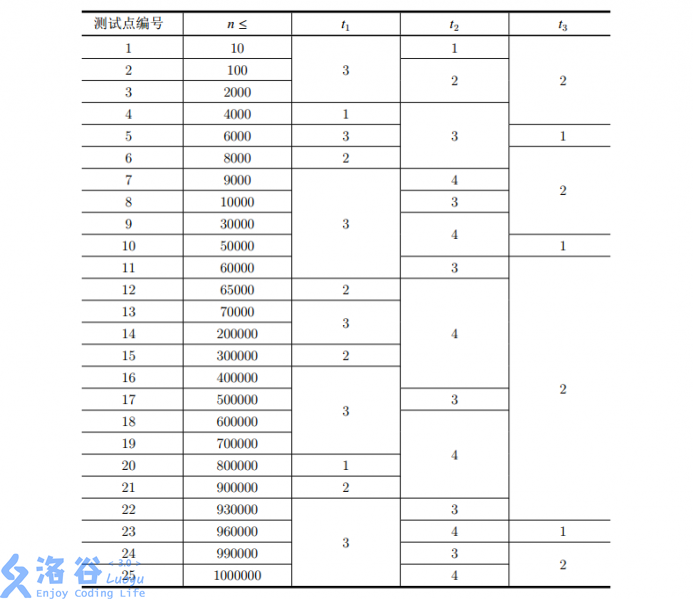P3822 [NOI2017]整数

• 474通过
• 2K提交
• 题目提供者 deluxurous
• 评测方式 云端评测
• 标签 线段树 进制 高精 NOI系列 2017 O2优化 高性能
• 难度 NOI/NOI+/CTSC
• 时空限制 2000ms / 512MB
• 提示：收藏到任务计划后，可在首页查看。

题目背景

在人类智慧的山巅，有着一台字长为$1048576$位（此数字与解题无关）的超级计算机，著名理论计算机科

学家P博士正用它进行各种研究。不幸的是，这天台风切断了电力系统，超级计算机

无法工作，而 P 博士明天就要交实验结果了，只好求助于学过OI的你. . . . . .

题目描述

P 博士将他的计算任务抽象为对一个整数的操作。

具体来说，有一个整数$x$，一开始为$0$。

接下来有$n$个操作，每个操作都是以下两种类型中的一种：

• 1 a b：将$x$加上整数$a\cdot 2^b$，其中$a$为一个整数，$b$为一个非负整数

• 2 k ：询问$x$在用二进制表示时，位权为$2^k$的位的值（即这一位上的$1$代表 $2^k$）

保证在任何时候，$x\geqslant 0$。

输入输出格式

输入格式：

输入的第一行包含四个正整数$n,t_1,t_2,t_3$，$n$的含义见题目描述，$t_1$，$t_2$，$t_3$的具体含义见子任务。

接下来$n$行，每行给出一个操作，具体格式和含义见题目描述。

同一行输入的相邻两个元素之间，用恰好一个空格隔开。

输出格式：

对于每个询问操作，输出一行，表示该询问的答案（$0$或$1$）。对于加法操作，没有任何输出。

输入输出样例

输入样例#1： 复制
10 3 1 2
1 100 0
1 2333 0
1 -233 0
2 5
2 7
2 15
1 5 15
2 15
1 -1 12
2 15
输出样例#1： 复制
0
1
0
1
0

说明

在所有测试点中，$1\leqslant t_1 \leqslant 3, 1 \leqslant t_2 \leqslant 4, 1 \leqslant t_3 \leqslant 2$。不同的 $t_1, t_2, t_3$ 对应的特殊限制如下：

• 对于 $t_1 = 1$ 的测试点，满足 $a = 1$

• 对于 $t_1 = 2$ 的测试点，满足 $|a| = 1$

• 对于 $t_1 = 3$ 的测试点，满足 $|a| \leqslant 10^9$

• 对于 $t_2 = 1$ 的测试点，满足 $0 \leqslant b, k \leqslant 30$

• 对于 $t_2 = 2$ 的测试点，满足 $0 \leqslant b, k \leqslant 100$

• 对于 $t_2 = 3$ 的测试点，满足 $0 \leqslant b, k \leqslant n$

• 对于 $t_2 = 4$ 的测试点，满足 $0 \leqslant b, k \leqslant 30n$

• 对于 $t_3 = 1$ 的测试点，保证所有询问操作都在所有修改操作之后

• 对于 $t_3 = 2$ 的测试点，不保证询问操作和修改操作的先后顺序

本题共 25 个测试点，每个测试点 4 分。各个测试点的数据范围如下：提示
标程仅供做题后或实在无思路时参考。
请自觉、自律地使用该功能并请对自己的学习负责。
如果发现恶意抄袭标程，将按照I类违反进行处理。